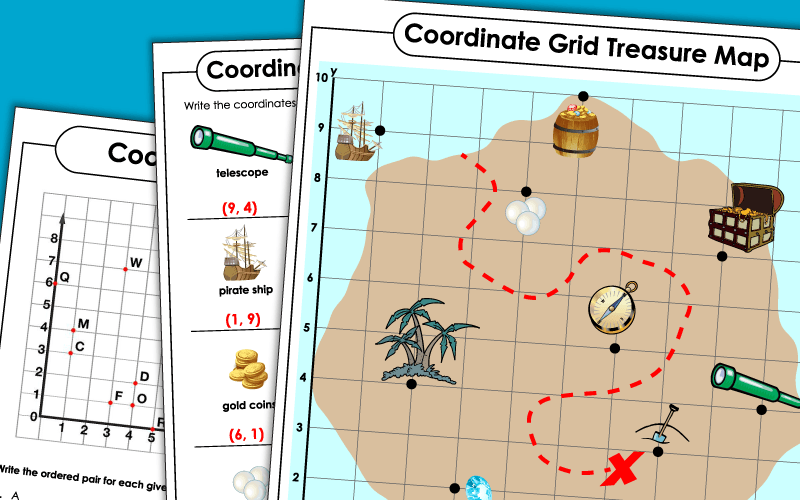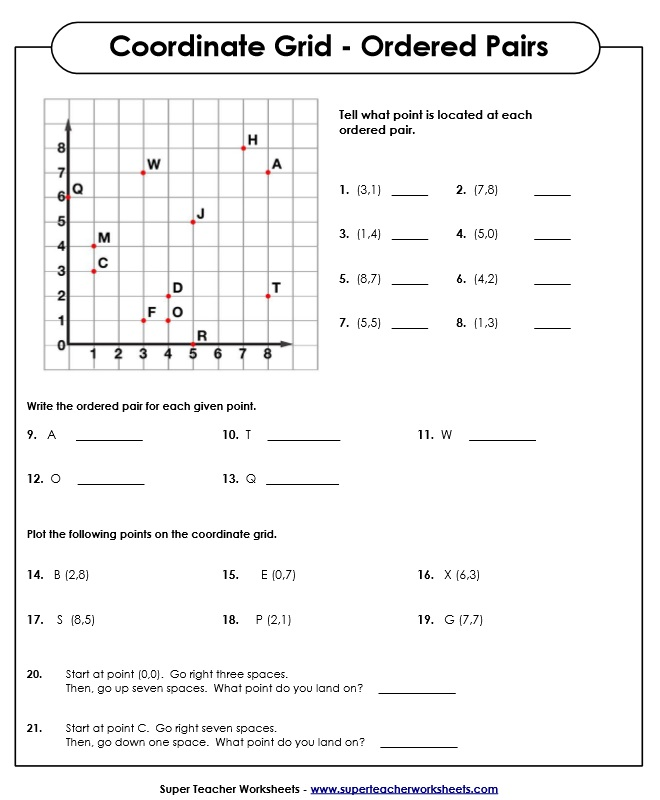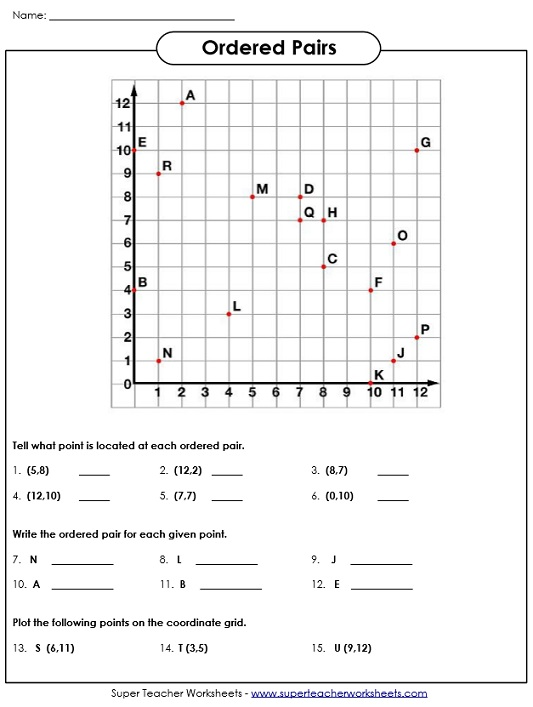# Ordered Pairs and Coordinate Plane Worksheets

Print out these worksheets on coordinate grids, coordinate planes, and ordered pairs.  Practice naming and plotting points on a grid.  Some worksheets include only positive numbers, while others include positive and negative numbers.## Basic(Positive Numbers to 20)

Identify the ordered pairs, then plot points on the coordinate grid.
More practice with ordered pairs on a coordinate plane.  This worksheet includes only positive numbers.
Using a single quadrant coordinate grid, students find the ordered pair of coordinates for pirate treasure.
Students list the ordered pairs for the vertices of a polygon on a single quadrant coordinate grid.
Tell what ordered pair is represented by the picture icons on the coordinate grid.  (Positive numbers only.)
On this worksheet, students use a chessboard's coordinate grid-like appearance to write the letter-number ordered pairs of various chess pieces.

## Intermediate(Positive Numbers to 100)

Students find the coordinates of treasure hidden on a pirate island, using a single quadrant coordinate grid.
Students identify and list the points on a single quadrant grid that form the vertices of a polygon. The numbers on the axes are scaled, making this an intermediate level worksheet.

Students use the coordinate grids to identify points, determine quadrant, and plot points.
Plot and find ordered pairs on a coordinate plane.  This worksheet includes both positive and negative numbers.
Students use the four quadrant coordinate grid treasure map to locate the coordinates of the pirate treasure.
Students chart the vertices of a polygon on a four quadrant coordinate grid.

## Also on Super Teacher Worksheets...

Mystery Graph Picture Worksheets

Plot the ordered pairs on the grid paper and connect lines to reveal graph art pictures.

Geometry Worksheets

Worksheets on polygons, perimeter, angles, area, lines, and more!

Graphing Worksheets

Printable bar graph worksheets.

## Pictures of Our WorksheetsMy Account
Site Information